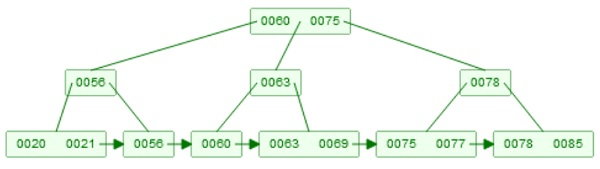# B+ tree Query in Data Structure

Here we will see, how to perform the searching in B+ Tree. The B+ Tree searching is also known as B+ Tree Querying. This algorithm is very much similar to the querying of B-Tree. Moreover, this supports range query. Suppose we have a B+ tree like below −

Example of B+ TreeThe searching technique is very similar to the binary search tree. Suppose we want to search 63 from the above tree. So we will start from root, now 63 is larger than root element 60 but smaller than 75. So we will move to the right child of the element 60. The right child is 63. But if we use B Tree, then that will be the result. In this case as the current node is internal node, then that is not an actual data. We have to move to the right child first. And at the leaf level, we can find the 63 as record. That will be the actual result.

if we want to search all elements from 63 to 78, then we do not need to backtrack each element, one by one. We find the leaf node of the initial value, then by using the linked leaf nodes, simply find all nodes before 78. So this supports range query.

Let us see the algorithm to search element inside the B-Tree.

## Algorithm

BPlusTreeSearch(root, key) −

Input − The root of the tree, and key to find

Output − The value of the node with key, if it is not present, return null

Apply binary search on records
if record with the ‘key’ is found, then
return required record
else if current node is leaf node, and key is not found, then
return null